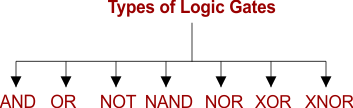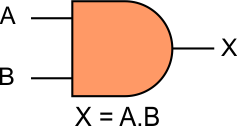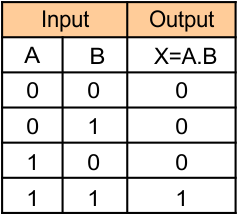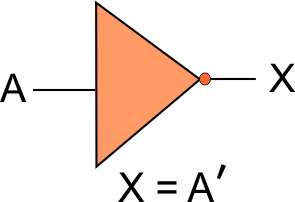Select Page

Computer Organization

Memory Organization
Interrupt
DMA Controller

# Logic Gates

The logic gates are an essential part of a digital system. The truth table is use to show the relationship between the input-output. Each gate has one or two binary input variables and one binary output variable. Binary input/output is in the form of 0 or 1.

## Types of Logic Gates

The seven basic logic gates includes: AND, OR, NOT, NOR, XOR, NAND, and XNOR. Every logic gate has a different representation and its operation. Let explain of these logic gates.### 1. AND GATE

The AND operation gives output only if all its inputs are 1 (ON). It is represented by a dot (.) symbol.

Notation of AND GATE

In AND operation, there are n inputs (n >= 2) and one output. If X is the output and A,B are the inputs then Boolean function of AND GATE can  be represented in any form of the following,

• X = A AND B
• X = A.B
• X = AB

Diagram of the AND GATE

##The Truth Table of AND GATE

##### 2. OR GATE

The OR operation gives output only if one or more of its inputs are 1 (ON). It is represented by a Plus (+) symbol.

Notation of OR GATE

In OR operation, there are n inputs (n >= 2) and one output. If X is the output and A,B are the inputs then Boolean function of OR Gate can  be represented in any form of the following,

• X = A OR B
• X = A + B

Diagram Of OR GATE

Truth Table Of OR GATE

### 3. NOT GATE

The NOT gate provide an inverted output of its input. i.e. if the input is 1 then the output will be 0 and vice versa. It also known as an Inverter.

Notation of NOT GATE

If X is the output and A is the inputs then Boleyn function can be represented in any form of the following,

• X = A-Bar
• X = NOT A
• X= A’

Diagram Of NOT GATETruth Table Of NOT GATE

### 4. NAND GATE

The NAND (NOT-AND) gate is equal to the combination of AND gate and NOT gate. NAND gate gives output (1) if any of the inputs are 0. The NAND gate symbolically represented as a AND gate with a small circle on the output. Notation of this small circle shows the inversion.

Notation of NAND GATE

In NAND operation there are n inputs (n >= 2) and one output. If X is the output and A,B are the inputs then Boleyn function can  be represented in any form of the following,

• X = (A AND B)’
• X = (A.B)’
• X = (AB)’
• X = (A.B)-Bar

Diagram of NAND GATE

Truth Table of NAND GATE

## 5. NOR GATE

NOR (NOT-OR) gate is equal to the combination of OR gate and NOT gate. It gives a 0 output if any of the inputs are 1. It is symbolically represented as an OR gate with a small circle on the output. Notation of this small circle shows the inversion.

Notation of NAND GATE

In NAND operation there are n inputs (n >= 2) and one output. If X is the output and A,B are the inputs then Boleyn function can  be represented in any form of the following,

• X = (A OR B)’
• X = (A+B)’
• X = A+B(BAR)

Diagram of NOR GATE

Truth Table of NOR GATE

### 6. Exclusive-OR/ XOR GATE

The XOR (‘Exclusive-OR’) gate gives an output (0) if both inputs are same i.e. A=0 and B=0. The XOR operation is denoted by an encircled plus sign ⊕.  The small narrow line at input side represents exclusive.

Notation of XOR GATE

Boolean function of XOR can be represented in any form of the following,

• X = A ⊕ B
• X = A’B+AB’

Diagram of XOR GATE

Truth Table of XOR GATE

### 7. Exclusive-NOR (X-NOR) GATE

The Exclusive-NOR gate applies the inversion operation on XOR gate. It will give an output (1) if both inputs are same i.e. A=0 and B=0. The small narrow line at input side represents exclusive. Small circle at output shows the inversion.

Notation Of X-NOR Gate

Boolean functions of Exclusive-NOR can be represented in any form of the following,

• X = (A ⊕ B)’
• X = A’B’+AB

Diagram of Exclusive-NOR

Truth Table of Exclusive-NOR GATE

## Combination Of Various Logic Gates

We can use various gates together to get the desired output i.e.one “OR gate” and Two “AND Gates”  as shown in the following diagram.

The truth table of  above diagram, given below

Help Other’s By Sharing…